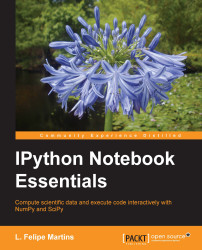•#### IPython Notebook Essentials#### Overview of this book

IPython Notebook EssentialsCreditswww.PacktPub.comPrefaceFree Chapter
A Tour of the IPython NotebookThe Notebook InterfaceGraphics with matplotlibHandling Data with pandasAdvanced Computing with SciPy, Numba, and NumbaProIPython Notebook Reference CardA Brief Review of PythonNumPy ArraysIndex## Summary

In this chapter, we covered the use of advanced mathematical algorithms in `SciPy`, including solving equations and finding optimal values, integration, and differential equations. The chapter concluded with a discussion on using parallelization in the GPU to accelerate computations.